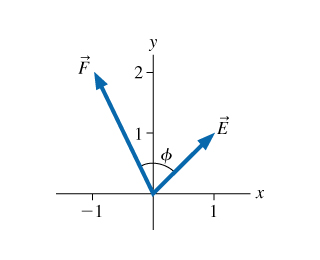# Problem: A. What is the angle ? between vectors E? and F? in the figure? (Figure 1)B. Use components to determine the magnitude of G?  =E? +F ?C. Use components to determine the direction of G?  =E? +F ?

###### FREE Expert Solution

Dot Product:

$\overline{)\stackrel{\mathbf{⇀}}{\mathbf{F}}{\mathbf{·}}\stackrel{\mathbf{⇀}}{\mathbf{E}}{\mathbf{=}}{\mathbf{F}}{\mathbf{E}}{\mathbf{c}}{\mathbf{o}}{\mathbf{s}}{\mathbf{\varphi }}}$

A.

From the dot product equation:

Φ = cos-1(F•E/FE)

F•E = (-i + 2j)•(i + j) = - 1 + 2 = 1

80% (359 ratings)###### Problem Details

A. What is the angle ? between vectors E? and F? in the figure? (Figure 1)

B. Use components to determine the magnitude of G?  =E? +?

C. Use components to determine the direction of G?  =E? +?